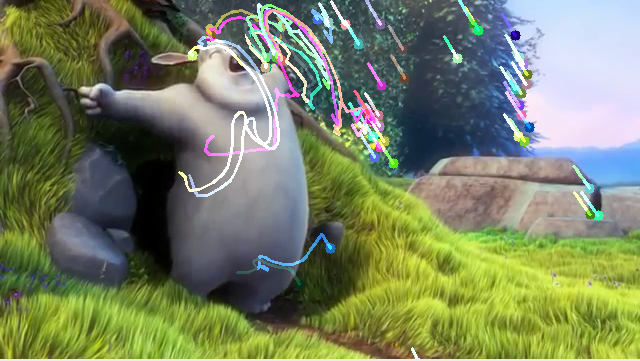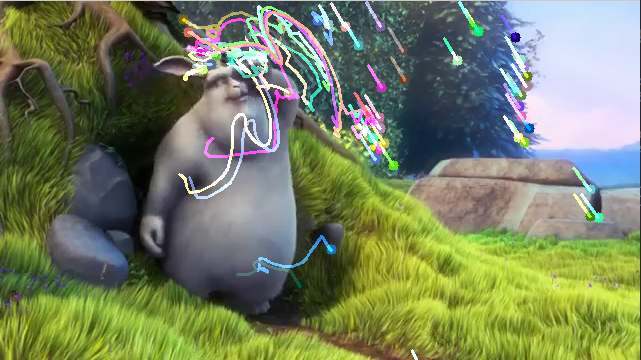Open in App
Not now

# Python OpenCV: Optical Flow with Lucas-Kanade method

• Difficulty Level : Expert
• Last Updated : 03 Jan, 2023

Prerequisites: OpenCV

OpenCV is a huge open-source library for computer vision, machine learning, and image processing. OpenCV supports a wide variety of programming languages like Python, C++, Java, etc. It can process images and videos to identify objects, faces, or even the handwriting of a human. When it is integrated with various libraries, such as Numpy which is a highly optimized library for numerical operations, then the number of weapons increases in your Arsenal i.e whatever operations one can do in Numpy can be combined with OpenCV.

In this article, we will be learning how to apply the Lucas-Kanade method to track some points on a video. To track the points, first, we need to find the points to be tracked. For finding the points, we’ll use `cv2.goodFeaturesToTrack()`. Now, we will capture the first frame and detect some corner points. These points will be tracked using the Lucas-Kanade Algorithm provided by OpenCV, i.e, `cv2.calcOpticalFlowPyrLK()`.

Syntax: cv2.calcOpticalFlowPyrLK(prevImg, nextImg, prevPts, nextPts[, winSize[, maxLevel[, criteria]]])

Parameters:
prevImg – first 8-bit input image
nextImg – second input image
prevPts – vector of 2D points for which the flow needs to be found.
winSize – size of the search window at each pyramid level.
maxLevel – 0-based maximal pyramid level number; if set to 0, pyramids are not used (single level), if set to 1, two levels are used, and so on.
criteria – parameter, specifying the termination criteria of the iterative search algorithm.

Return:
nextPts – output vector of 2D points (with single-precision floating-point coordinates) containing the calculated new positions of input features in the second image; when OPTFLOW_USE_INITIAL_FLOW flag is passed, the vector must have the same size as in the input.
status – output status vector (of unsigned chars); each element of the vector is set to 1 if the flow for the corresponding features has been found, otherwise, it is set to 0.
err – output vector of errors; each element of the vector is set to an error for the corresponding feature, type of the error measure can be set in flags parameter; if the flow wasn’t found then the error is not defined (use the status parameter to find such cases).

Example:

 `import` `numpy as np ` `import` `cv2 ` ` `  `cap ``=` `cv2.VideoCapture(``'sample.mp4'``) ` ` `  `# params for corner detection ` `feature_params ``=` `dict``( maxCorners ``=` `100``, ` `                       ``qualityLevel ``=` `0.3``, ` `                       ``minDistance ``=` `7``, ` `                       ``blockSize ``=` `7` `) ` ` `  `# Parameters for lucas kanade optical flow ` `lk_params ``=` `dict``( winSize ``=` `(``15``, ``15``), ` `                  ``maxLevel ``=` `2``, ` `                  ``criteria ``=` `(cv2.TERM_CRITERIA_EPS | cv2.TERM_CRITERIA_COUNT, ` `                              ``10``, ``0.03``)) ` ` `  `# Create some random colors ` `color ``=` `np.random.randint(``0``, ``255``, (``100``, ``3``)) ` ` `  `# Take first frame and find corners in it ` `ret, old_frame ``=` `cap.read() ` `old_gray ``=` `cv2.cvtColor(old_frame, ` `                        ``cv2.COLOR_BGR2GRAY) ` `p0 ``=` `cv2.goodFeaturesToTrack(old_gray, mask ``=` `None``, ` `                             ``*``*``feature_params) ` ` `  `# Create a mask image for drawing purposes ` `mask ``=` `np.zeros_like(old_frame) ` ` `  `while``(``1``): ` `     `  `    ``ret, frame ``=` `cap.read() ` `    ``frame_gray ``=` `cv2.cvtColor(frame, ` `                              ``cv2.COLOR_BGR2GRAY) ` ` `  `    ``# calculate optical flow ` `    ``p1, st, err ``=` `cv2.calcOpticalFlowPyrLK(old_gray, ` `                                           ``frame_gray, ` `                                           ``p0, ``None``, ` `                                           ``*``*``lk_params) ` ` `  `    ``# Select good points ` `    ``good_new ``=` `p1[st ``=``=` `1``] ` `    ``good_old ``=` `p0[st ``=``=` `1``] ` ` `  `    ``# draw the tracks ` `    ``for` `i, (new, old) ``in` `enumerate``(``zip``(good_new,  ` `                                       ``good_old)): ` `        ``a, b ``=` `new.ravel() ` `        ``c, d ``=` `old.ravel() ` `        ``mask ``=` `cv2.line(mask, (a, b), (c, d), ` `                        ``color[i].tolist(), ``2``) ` `         `  `        ``frame ``=` `cv2.circle(frame, (a, b), ``5``, ` `                           ``color[i].tolist(), ``-``1``) ` `         `  `    ``img ``=` `cv2.add(frame, mask) ` ` `  `    ``cv2.imshow(``'frame'``, img) ` `     `  `    ``k ``=` `cv2.waitKey(``25``) ` `    ``if` `k ``=``=` `27``: ` `        ``break` ` `  `    ``# Updating Previous frame and points  ` `    ``old_gray ``=` `frame_gray.copy() ` `    ``p0 ``=` `good_new.reshape(``-``1``, ``1``, ``2``) ` ` `  `cv2.destroyAllWindows() ` `cap.release() `

Output:My Personal Notes arrow_drop_up
Related Articles